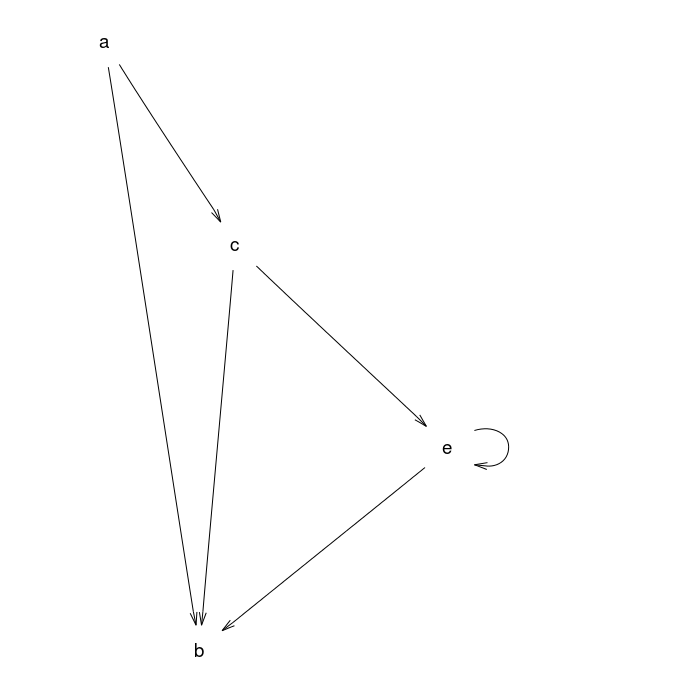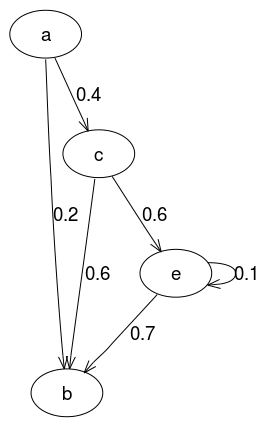# Example 4: "full" directed graph

A "full" directed graph with four nodes and four labelled, weighted edges.

## Graph declaration

In all cases the graph is explicitly declared with ellipse shaped nodes. The dot file edges are declared in alphabetical order as this is the (assumed) default for how Rgraphviz will pass edges internally to graphviz.

 Dot file for graphviz: `````` digraph { node [shape=ellipse]; // nodes a b c e; // labelled, weighted edges a -> b[label="0.2",weight="0.2"]; a -> c[label="0.4",weight="0.4"]; c -> b[label="0.6",weight="0.6"]; c -> e[label="0.6",weight="0.6"]; e -> e[label="0.1",weight="0.1"]; e -> b[label="0.7",weight="0.7"]; } `````` R code for Rgraphviz and gridGraphviz: ```# load Rgraphviz library library(Rgraphviz) # nodes nodes <- c("a", "b", "c", "e") # edges with weights edgeList <- list( a = list(edges = c("b", "c"), weights = c(0.2, 0.4)), b = list(), c = list(edges = c("b", "e"), weights = c(0.6, 0.6)), e = list(edges = c("b", "e"), weights = c(0.7, 0.1))) graph <- new("graphNEL", nodes = nodes, edgeL = edgeList, edgemode = "directed") # use edge weights as edge labels labels <- unlist(edgeWeights(graph)) names(labels) <- edgeNames(graph) ```

## Graph rendering:

### gridGraphviz (new)

``````>> dot -Tpng ex4.dot -o ex4.png
`````````# create Ragraph object
rag <- agopen(graph, "", attrs=list(node=list(shape="ellipse")),
edgeAttrs=list(label=labels))
```
```#plot
plot(rag)
``````library(gridGraphviz)

# create Ragraph object
rag <- agopen(graph, "", attrs=list(node=list(shape="ellipse")),
edgeAttrs=list(label=labels))
```
```#plot
grid.graph(rag)
```
```## Warning: Unsupported node shape; using 'box'
## Warning: Unsupported node shape; using 'box'
## Warning: Unsupported node shape; using 'box'
## Warning: Unsupported node shape; using 'box'
``````library(gridGraphviz)

# create Ragraph object
rag <- agopenTrue(graph, "", attrs=list(node=list(shape="ellipse")),
edgeAttrs=list(label=labels))
```
```#plot
grid.graph(rag)
```## Discussion

gridGraphviz (old) fails to produce ellipse shaped nodes, and to display edge labels.